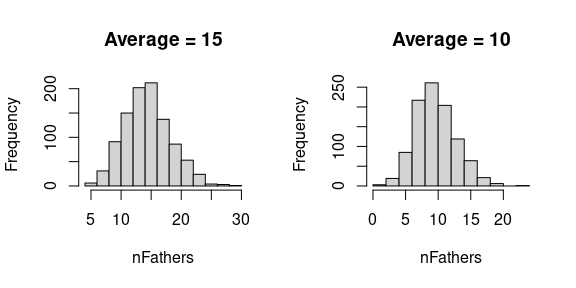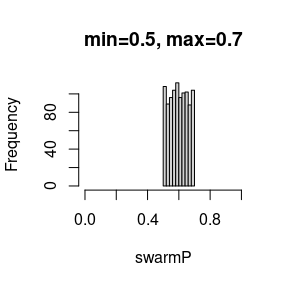# Introduction

SIMplyBee includes functions to sample various values that are expected to vary between colonies and events. These functions are used to sample numbers, usually individuals, and proportions. We can use the functions, pass them to other functions, or save them in the SimParamBee object so they can be used by default by other functions.

library(package = "SIMplyBee")

# Functions to sample numbers

First, there are functions to sample the number of caste individuals from either a Poisson or truncated Poisson distribution: n*Poisson() and n*TruncPoisson(), where * is either Workers, Drones, VirginQueens, or Fathers. Most SIMplyBee functions that take the number of individuals as an argument can accept these sampling functions as an input, meaning that the output of such function calls will be stochastic. These functions are useful when you want to sample a variable number of individuals around a mean, as for example when mating virgin queens with a variable number of drones.

Let’s start a simulation by creating a DCA and an apiary with 10 virgin colonies:

founderGenomes <- quickHaplo(nInd = 20, nChr = 1, segSites = 100)
SP <- SimParamBee$new(founderGenomes) basePop <- createVirginQueens(founderGenomes) # Create a DCA from the first 10 base virgin queens DCA <- createDrones(x = basePop[1:10], nInd = 100) # Create an apiary with 10 virgin colonies apiary <- createMultiColony(basePop[11:20]) From the literature we know that virgin queens on average mate to 17 drones, but the actual number varies around this mean. Some mate with 10 drones, some with 20, etc. To resemble this variation, we can use the function nFathersPoisson() to sample variable number of drones from a DCA. The default average for this function is 15, but you can use any value you want. Let’s use this function to sample 1,000 values and inspect the distribution and the mean.Let’s now use this functionality to sample a variable number of drones from the DCA to mate with each of the 10 virgin queens. droneGroups <- pullDroneGroupsFromDCA(DCA = DCA, n = 10, nDrones = nFathersPoisson) apiary <- cross(apiary, drones = droneGroups) And inspect the number of fathers in each of the colony and their mean. nFathers(apiary) #> 1 2 3 4 5 6 7 8 9 10 #> 15 13 11 15 19 15 18 23 14 16 mean(nFathers(apiary)) #>  15.9 Second, we have a group of functions that will sample the number of individuals according to the colony phenotype, whatever that might be. These functions are named n*ColonyPhenotype(), where * is either is either Workers, Drones, or VirginQueens. An example of this would be sampling the number of workers and drones according to queen’s fecundity or honey yield. An example of this can be seen in the quantitative genetics vignette in the “Strength and honey yield” example. # Functions that sample proportions SIMplyBee also includes functions to sample the proportions of workers that leave or are removed when downsizing, splitting, or swarming a colony from either a uniform distribution or from a beta distribution that accounts for the number of individuals in a colony (colony strength). These functions are named *PUnif(), where * can be either swarm, split, or downsize. There is an additional function, splitPColonyStrength(), that determines the number of workers to be removed in a split according to the colony strength. Let’s say we want to swarm all the colonies in our apiary with a variable percentage of workers that leave. We want to sample this percentage from an uniform distribution with the mean of 0.6. For this, we use swarmPUnif() function that takes a min and a max values and sample a value between them. By default, the min is set to 0.4 and max to 0.6. Let’s use this function to sample a 1,000 values between 0.5 and 0.7 and inspect the mean.Let’s now swarm all the colonies in our apiary with a variable percentage of workers that leave. apiary <- buildUp(apiary, nWorkers = 1000, nDrones = 100) tmp <- swarm(apiary, p = swarmPUnif(n = 10, min = 0.5, max = 0.7)) nWorkers(tmp$swarm)
#>  11  13  15  17  19  21  23  25  27  29
#> 642 640 605 538 657 573 539 695 628 546

We see that each colony swarmed with a different percentage between 0.5 and 0.7.